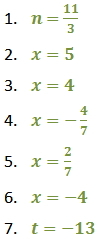Solve Equations with a Variable on One Side of the Equation

Solve Equations with a Variable on One Side of the Equation

To solve linear equations, isolate the variable, or get the variable alone on one side of the equals sign.

Steps:

1. If the equation contains fractions, you may choose to multiply both sides of the equation by the least common denominator (LCD). This will eliminate fractions from your equation.

2. Use the distributive property to remove parentheses.

3. Combine like terms on the same side of the equals sign.

4. Use the addition property to get the variable on one side and constants on the other.

5. Use the multiplication property to isolate the variable.

6. Check the solution by plugging your answer into the original equation.

Example 1 - Solve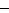2x5 =3

First add 5 to both sides2x5 + 53 + 5

Then multiply both sides by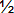2x(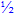) = 2()

x1

Example 2 - Solve for the following expression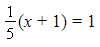Multiply both sides by the LCD, 5.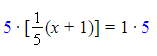x + 1 = 5

x = 4

Practice

Solve the following equations.

1) 5n - 2(n + 4) = 3

2) 16 = 4x + 6 - 2x

3) x + 1.24 - 0.07x = 4.96

4)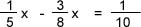5) 1 + (3 + x) + 6x = 6

6) 3(x - 2) - 4(2x + 3) = 2

7) 1/3 (t - 5) = -6